Examples

Chapter 12 Class 9 Herons Formula
Serial order wise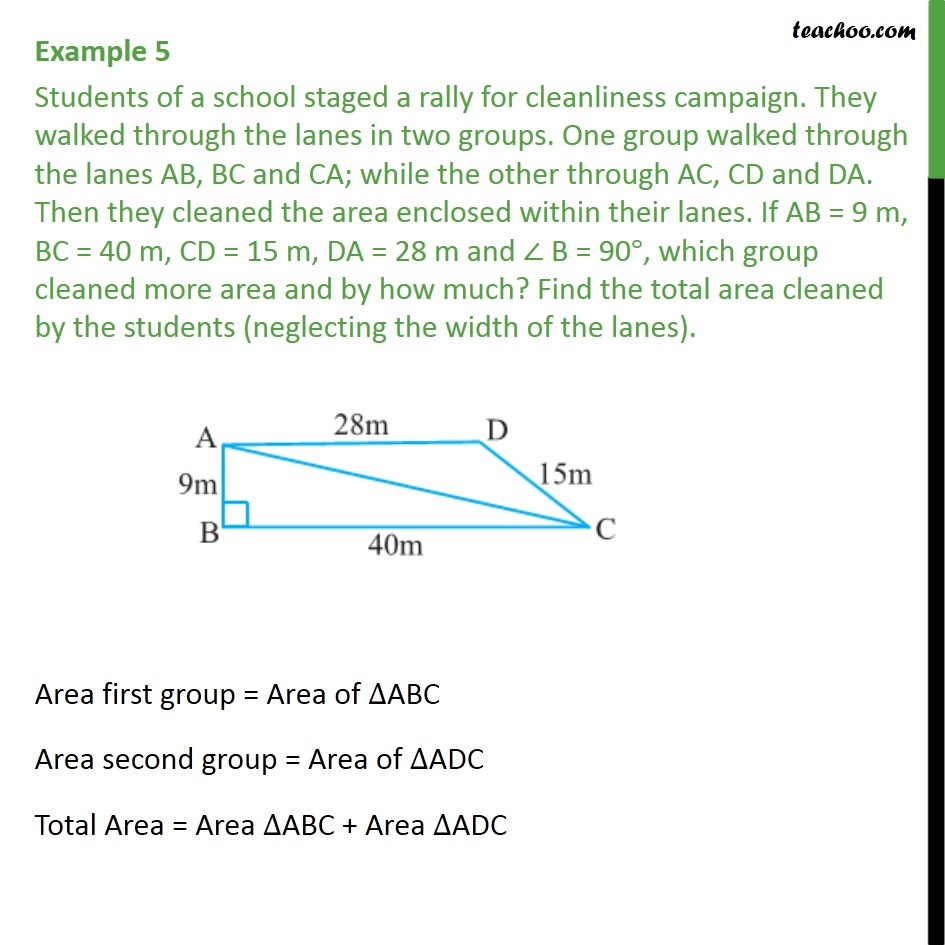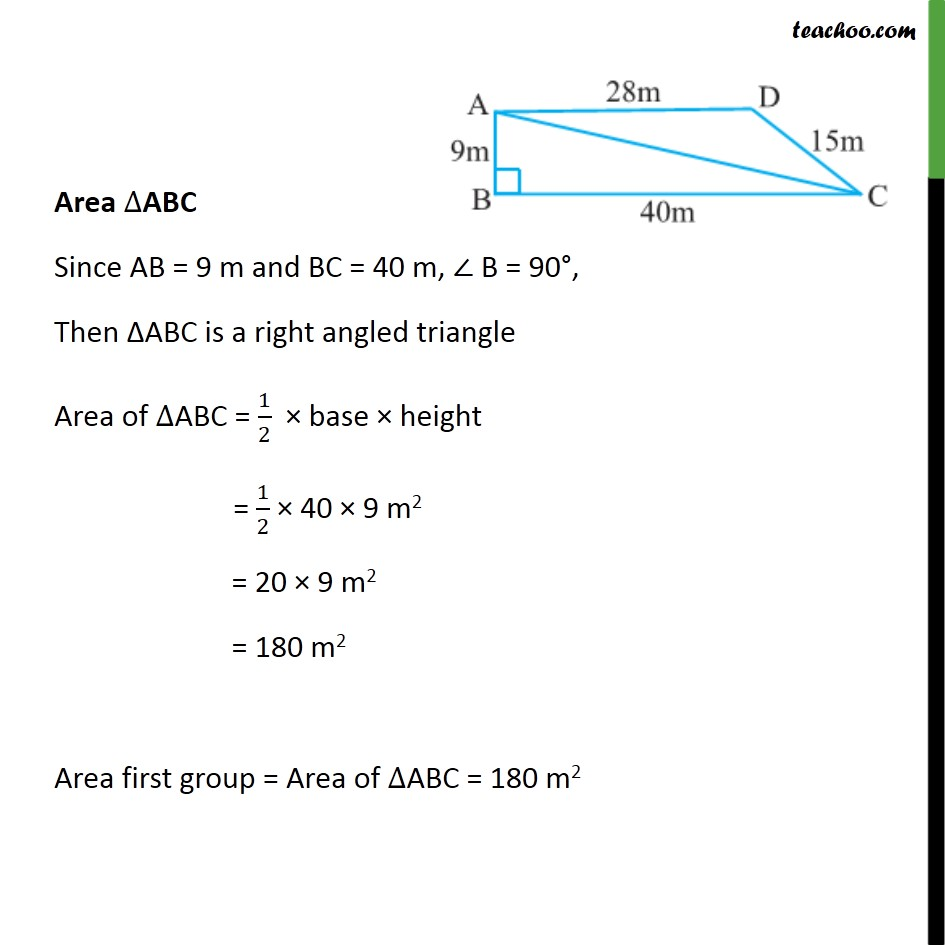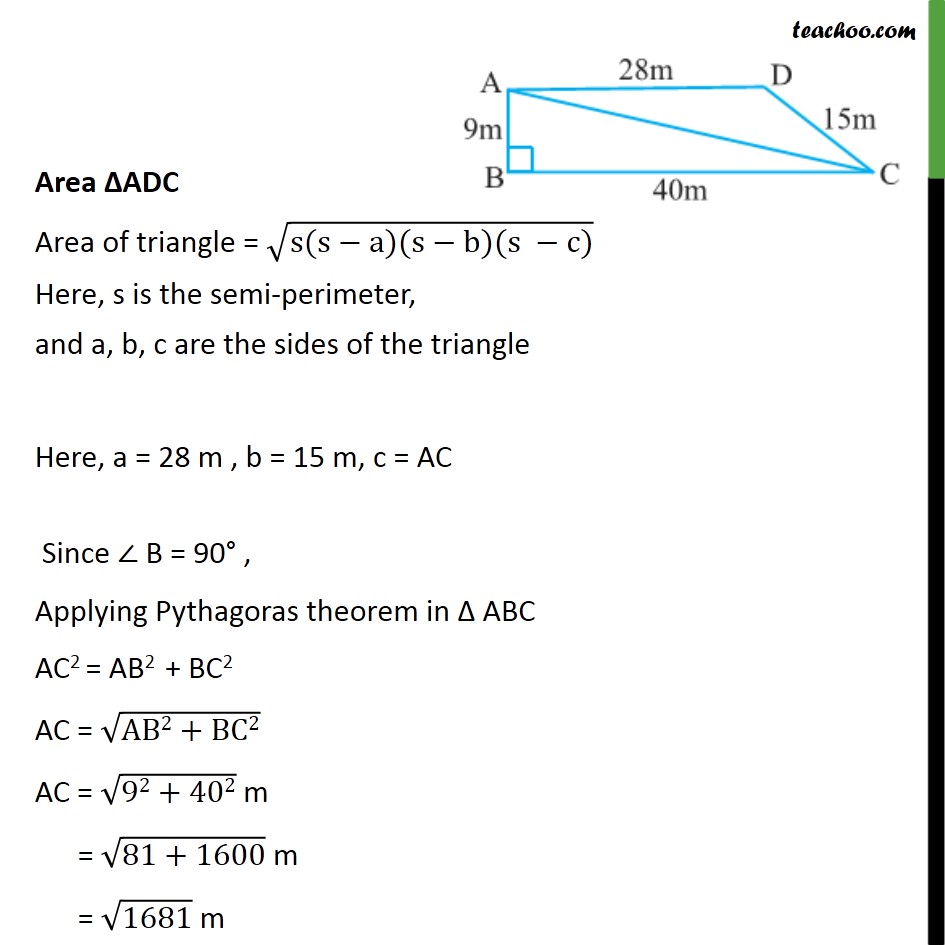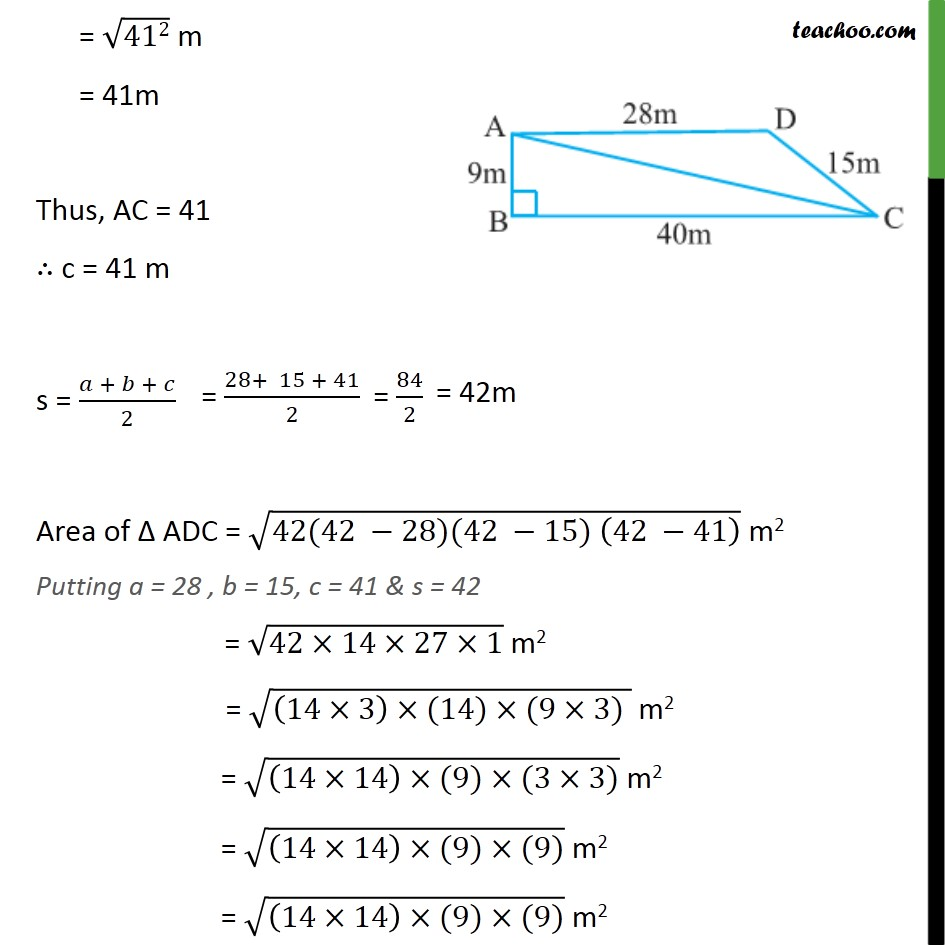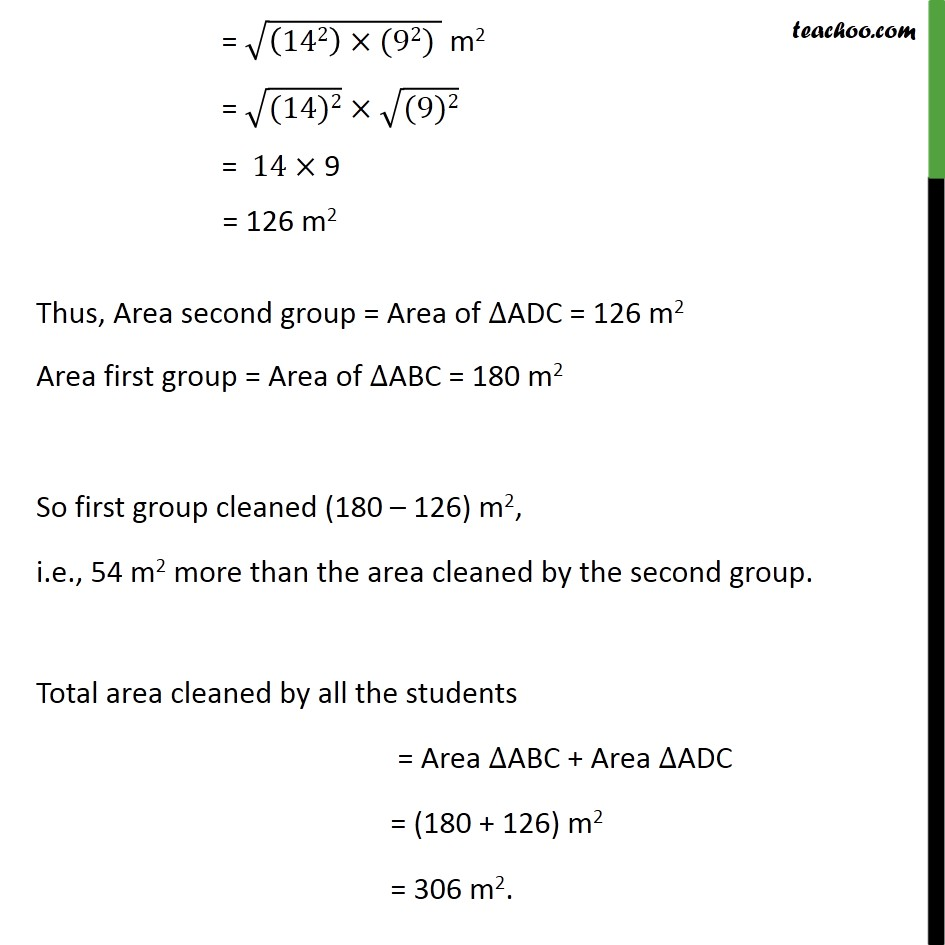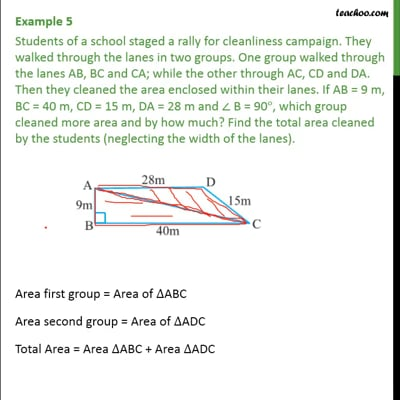This video is only available for Teachoo black users

Solve all your doubts with Teachoo Black (new monthly pack available now!)

### Transcript

Example 5 Students of a school staged a rally for cleanliness campaign. They walked through the lanes in two groups. One group walked through the lanes AB, BC and CA; while the other through AC, CD and DA. Then they cleaned the area enclosed within their lanes. If AB = 9 m, BC = 40 m, CD = 15 m, DA = 28 m and ∠ B = 90°, which group cleaned more area and by how much? Find the total area cleaned by the students (neglecting the width of the lanes). Area first group = Area of ∆ABC Area second group = Area of ∆ADC Total Area = Area ∆ABC + Area ∆ADC Area ∆ABC Since AB = 9 m and BC = 40 m, ∠ B = 90°, Then ΔABC is a right angled triangle Area of ∆ABC = 1/2 × base × height = 1/2 × 40 × 9 m2 = 20 × 9 m2 = 180 m2 Area first group = Area of ∆ABC = 180 m2 Area ΔADC Area of triangle = √(s(s−a)(s−b)(s −c)) Here, s is the semi-perimeter, and a, b, c are the sides of the triangle Here, a = 28 m , b = 15 m, c = AC Since ∠ B = 90° , Applying Pythagoras theorem in Δ ABC AC2 = AB2 + BC2 AC = √(AB2+BC2) AC = √(92+402) m = √(81+1600) m = √1681 m = √412 m = 41m Thus, AC = 41 ∴ c = 41 m s = (𝑎 + 𝑏 + 𝑐)/2 Area of Δ ADC = √(42(42 −28)(42 −15) (42 −41) ) m2 Putting a = 28 , b = 15, c = 41 & s = 42 = √(42×14×27×1) m2 = √((14×3)×(14)×(9×3) ) m2 = √((14×14)×(9)×(3×3)) m2 = √((14×14)×(9)×(9)) m2 = √((14×14)×(9)×(9)) m2 = √((142)×(92) ) m2 = √((14)2) × √((9)2) = 14× 9 = 126 m2 Thus, Area second group = Area of ∆ADC = 126 m2 Area first group = Area of ∆ABC = 180 m2 So first group cleaned (180 – 126) m2, i.e., 54 m2 more than the area cleaned by the second group. Total area cleaned by all the students = Area ∆ABC + Area ∆ADC = (180 + 126) m2 = 306 m2.